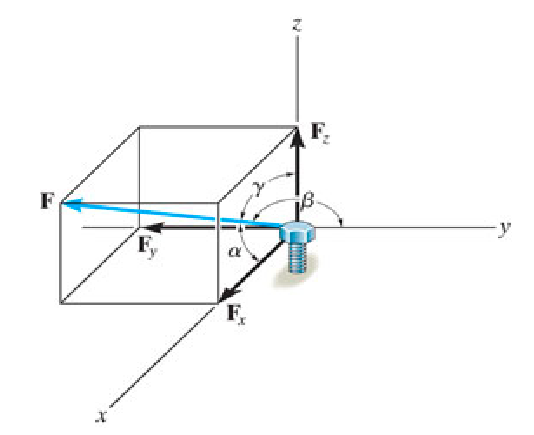# Problem: The bolt is subjected to the force F, which has components acting along the x, y, z axes as shown.Part AIf the magnitude of F is 90 N , and α = 64° and γ = 41°, determine the magnitude of Fx component.Part BDetermine the magnitude of Fy component.Part CDetermine the magnitude of Fz component.

###### FREE Expert Solution

We are given a 3D force. We'll need various relations.

$\overline{){{\mathbf{\left(}}{\mathbf{c}}{\mathbf{o}}{\mathbf{s}}{\mathbf{\alpha }}{\mathbf{\right)}}}^{{\mathbf{2}}}{\mathbf{+}}{{\mathbf{\left(}}{\mathbf{c}}{\mathbf{o}}{\mathbf{s}}{\mathbf{\beta }}{\mathbf{\right)}}}^{{\mathbf{2}}}{\mathbf{+}}{{\mathbf{\left(}}{\mathbf{c}}{\mathbf{o}}{\mathbf{s}}{\mathbf{\gamma }}{\mathbf{\right)}}}^{{\mathbf{2}}}{\mathbf{=}}{\mathbf{1}}}$

Part A

The x-component of force is:

$\overline{){{\mathbf{F}}}_{{\mathbf{x}}}{\mathbf{=}}{\mathbf{F}}{\mathbf{c}}{\mathbf{o}}{\mathbf{s}}{\mathbf{\alpha }}}$

We're given the magnitude of force to be 90N and α = 64°

99% (294 ratings)###### Problem DetailsThe bolt is subjected to the force F, which has components acting along the x, y, z axes as shown.
Part A

If the magnitude of F is 90 N , and α = 64° and γ = 41°, determine the magnitude of Fx component.

Part B

Determine the magnitude of Fcomponent.

Part C

Determine the magnitude of Fz component.

Frequently Asked Questions

What scientific concept do you need to know in order to solve this problem?

Our tutors have indicated that to solve this problem you will need to apply the Vectors in 3D concept. If you need more Vectors in 3D practice, you can also practice Vectors in 3D practice problems.